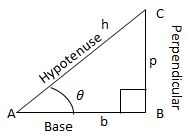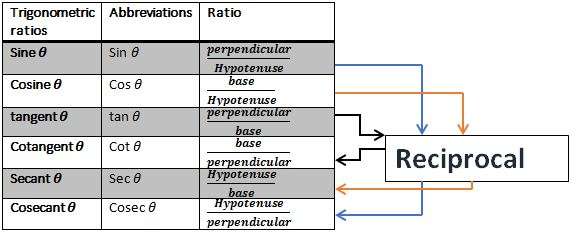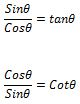## Revision Notes for Ch 8 Introduction to Trigonometry Class 10th Mathematics

Trigonometry and Trigonometric Ratios

→ Trigonometry is the branch of mathematics which deals with measurements of angles and the problems related to relationship between sides and angles.

Trigonometric Ratios:

→ It is the ratios of two sides of a right angle triangle.

→ Suppose we have a right angled triangle ABC.→ Thus the trigonometric Ratios are defined as the largest side of a right angle triangle is known as hypotenuse.

Also, by Pythagoras theorem, we know that h2 =b2+p2.

Here, θ is pronounced as theta, ∠A=θ. We write trigonometric ratios according to angle θ.Trigonometric Ratios for Few Particular AngelsNote:- Some Angles like cosecθ for θ=0° is not defined. We know that cosecθ = 1/sinθ = 1/0. 1/0 is not defined hence cosec0° is not defined.

Trigonometric Identities

→ Identities are equations which are valid for any conditions. Trigonometric identities are equations involving trigonometric ratios.

Some Trigonometric Identities are:

(i) sin2θ+cos2⁡θ=1(iv) sec2θ-tan2θ=1

(v) cosec2θ-cot2⁡θ=1

Trigonometric Ratios for Complementary Angles

(i) sin (90 – θ) = cosθ
(ii) cos (90 – θ) = sinθ
(iii) tan (90 – θ) = cotθ
(iv) cot (90 – θ) = tanθ
(v) sec (90 – θ) = cosecθ
(vi) cosec (90 – θ) = secθ